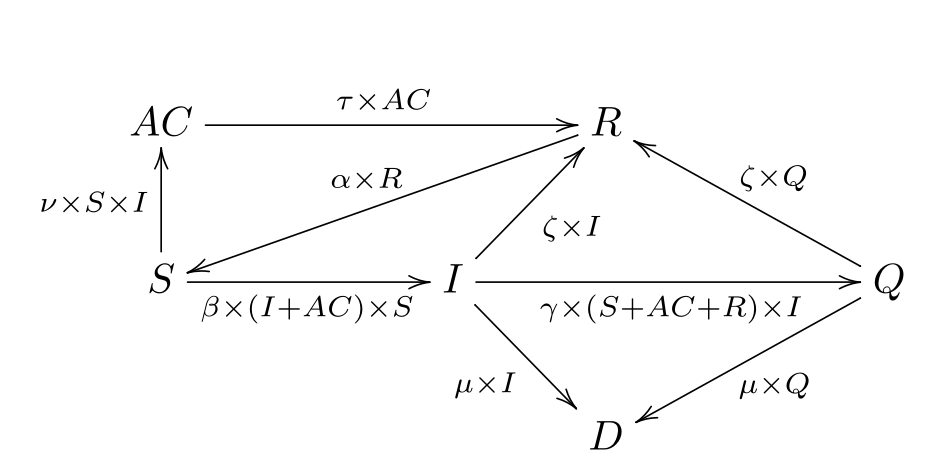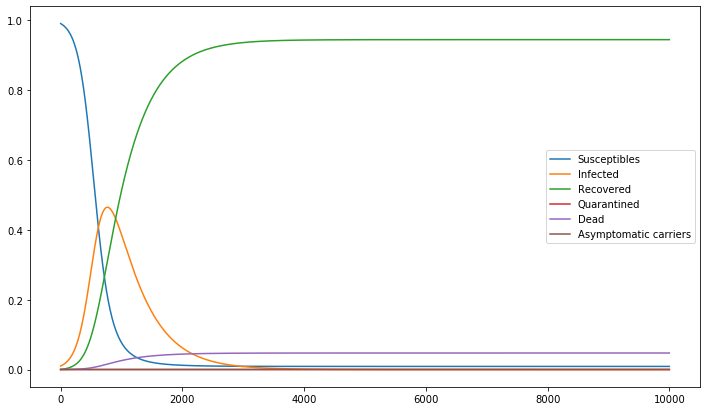### Zombie propagation

###### Sat 21 March 2020
Zombie favorite food warning (Photo credit: wikipedia)

I recently read a paper  trying to model a disease propagation. I wanted to play with this model.

## The model

The model is know as "SIR" as it divide the population into 3 groups:

• S: suceptible to become a zombie
• I: infected, and thus zombified
• R: recovered, and (hopefully) immune

It then model the move of individuals between groups. The principle of dividing a population into such group and modeling the transitions of individual is known as a compartmental model and many results have already been published about such models. I won't insist on the $$R_0$$ (basic reproduction number).

For fun, and because it is already done by scientific researchers, I will add other groups:

• Q: quarantined
• AC: asymptomatic carrier (exposed, can infect other but not zombified)

I'll use the follwing transition between groups:Zombie transition diagram

Note that:

• this is only a toy model
• transitions rates are proportional to population of some groups (more zombies and more suceptibles $$\implies$$ more mettings between both groups, zombies can be put in quarantine faster if there is more non-zombie able to do it)
• $$\zeta = \frac{1}{\lambda}$$ in some research paper, $$\lambda$$ being a recovery time.
• only zombie can die, e.i. other death causes are not modeled
• once recovered, there is a slight chance to not be immune (model either mutation in the desease or people needing a booster shot)

This model is thus entirely described by the following equations:

\begin{equation*} \begin{cases} \frac{\partial{S}}{\partial{t}} &= - \nu S(t) I(t) + \alpha R(t) - \beta (I(t) + AC(t)) \times S(t)\\ \frac{\partial{I}}{\partial{t}} &= \beta (I(t) + AC(t)) \times S(t) - \zeta I(t) - \gamma (S(t) + AC(t) + R(t)) \times I(t) - \mu I(t)\\ \frac{\partial{R}}{\partial{t}} &= \zeta (I(t) + Q(t)) + \tau AC(t) - \alpha R(t)\\ \frac{\partial{Q}}{\partial{t}} &= \gamma (S(t) + AC(t) + R(t)) \times I(t) - (\mu + \zeta) Q(t)\\ \frac{\partial{D}}{\partial{t}} &= \mu(I(t) + Q(t))\\ \frac{\partial{AC}}{\partial{t}} &= \nu S(t) I(t) - \tau AC(t) \end{cases} \end{equation*}

## The game

I use python to code this model. I use scipy.integrate.odeint. I simulated some scenarii 

This model clearly needs improvements (quarantine is useless in this model). In this model the asymptomous carrier, even if they are few, can have a visible influence on the outcome.

We can now collect possible outcomes:C Witkowski and B Blais (2013), "Bayesian analysis of epidemics-zombies, influenza, and other diseases"
  a jupyter notebook containing my code is available here (also in pdf)

Related articles (or not):

Category: maths Tagged: python maths zombie